0
170

# Geometry Triangles Questions for SSC MTS

Here you can download the Geometry Triangles Questions for SSC MTS PDF with solutions by Cracku. These are the most important Geometry Triangles questions PDF prepared by various sources also based on previous year’s papers. Utilize this PDF to Geometry Triangles for SSC MTS. You can find a list of Geometry Triangles in this PDF which help you to test yourself and practice. So you can click on the below link to download the PDF for reference and do more practice.

Question 1: $\triangle$ABC $\sim$ $\triangle$DEF and the area of $\triangle$ABC is 13.5 cm$^2$ and the area of $\triangle$DEF is 24 cm$^2$ . If BC = 3.15 cm, then the length (in cm) of EF is:

a) 4.8

b) 3.9

c) 5.1

d) 4.2

Solution: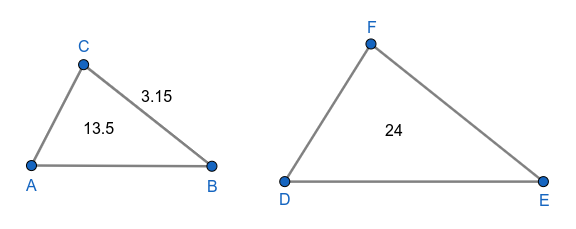$\triangle$ABC $\sim$ $\triangle$DEF

$\Rightarrow$  $\frac{\text{Area of triangle ABC}}{\text{Area of triangle DEF}}$ = $\left(\frac{BC}{EF}\right)^2$

$\Rightarrow$  $\frac{13.5}{24}=\left(\frac{3.15}{EF}\right)^2$

$\Rightarrow$  $\frac{135}{240}=\left(\frac{3.15}{EF}\right)^2$

$\Rightarrow$  $\frac{9}{16}=\left(\frac{3.15}{EF}\right)^2$

$\Rightarrow$  $\frac{3.15}{EF}=\frac{3}{4}$

$\Rightarrow$  EF = 4.2 cm

Hence, the correct answer is Option D

Question 2: In $\triangle$ABC, $\angle$C = 90$^\circ$ and Q is the midpoint of BC. If AB = 10 cm and AC = $2\sqrt{10}$ cm, then the length of AQ is:

a) $\sqrt{55}$ cm

b) $5\sqrt{3}$ cm

c) $5\sqrt{2}$ cm

d) $3\sqrt{5}$ cm

Solution: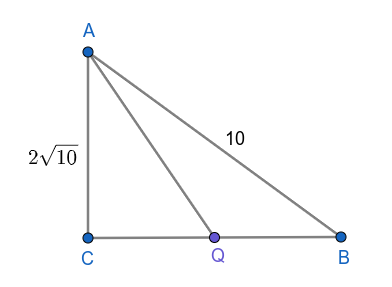From right angled triangle ABC,

AC$^2$ + BC$^2$ = AB$^2$

$\left(2\sqrt{10}\right)^2$ + BC$^2$ = $\left(10\right)^2$

40 + BC$^2$ = 100

BC$^2$ = 60

BC = $2\sqrt{15}$ cm

Q is the midpoint of BC.

CQ = $\frac{BC}{2}$ = $\sqrt{15}$ cm

From right angled triangle ACQ,

AC$^2$ + CQ$^2$ = AQ$^2$

$\left(2\sqrt{10}\right)^2$ + $\left(\sqrt{15}\right)^2$ = AQ$^2$

40 + 15 = AQ$^2$

AQ$^2$ = 55

AQ = $\sqrt{55}$ cm

Hence, the correct answer is Option A

Question 3: In $\triangle ABC$ and $\triangle DEF$ we have $\frac{AB}{DF} = \frac{BC}{DE} = \frac{AC}{EF}$, then which of the following is true?

a) $\triangle BCA \sim \triangle DEF$

b) $\triangle DEF \sim \triangle ABC$

c) $\triangle DEF \sim \triangle BAC$

d) $\triangle CAB \sim \triangle DEF$

Solution: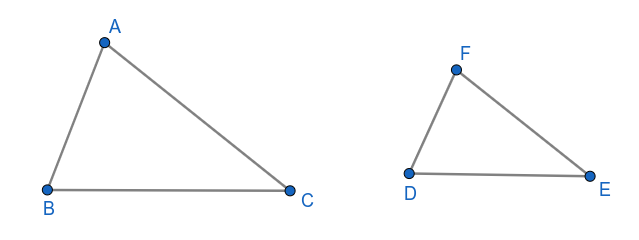Hence, the correct answer is Option A

Question 4: In a triangle ABC, length of the side AC is 4 cm more than 2 times the length of the side AB. Length of the side BC is 4 cm less than the three times the length of the side AB. If the perimeter of $\triangle$ABC is 60 cm, then its area (in cm$^2$) is:

a) 150

b) 144

c) 100

d) 120

Solution:

Length of the side AC is 4 cm more than 2 times the length of the side AB.

b = 2c + 4……(1)

Length of the side BC is 4 cm less than the three times the length of the side AB.

a = 3c – 4…….(2)

Perimeter of $\triangle$ABC is 60 cm.

a + b + c = 60

3c – 4 + 2c + 4 + c = 60

6c = 60

c = 10 cm

b = 2c + 4 = 24 cm

a = 3c – 4 = 26 cm

Half of the perimeter(s) = 30 cm

Area of the triangle = $\sqrt{s\left(s-a\right)\left(s-b\right)\left(s-c\right)}$

= $\sqrt{30\left(30-26\right)\left(30-24\right)\left(30-10\right)}$

= $\sqrt{30\left(4\right)\left(6\right)\left(20\right)}$

= $\sqrt{120\times120}$

= 120 cm$^2$

Hence, the correct answer is Option D

Question 5: The area of a table top in the shape of an equilateral triangle is $9\sqrt{3}$ cm$^2$. What is the length (in cm) of each side of the table?

a) 6

b) 2

c) 4

d) 3

Solution:

Let the length of each side of the table = a

The area of a table top in the shape of an equilateral triangle is $9\sqrt{3}$ cm$^2$.

$\frac{\sqrt{3}}{4}$a$^2$ = $9\sqrt{3}$

a$^2$ = 36

a = 6 cm

Length of each side of the table = 6 cm

Hence, the correct answer is Option A

Question 6: Let $\triangle$ABC $\sim$ $\triangle$RPQ and $\frac{ar(\triangle \text{ABC})}{ar(\triangle \text{PQR})}=\frac{16}{25}$. If PQ = 4 cm, QR = 6 cm and PR = 7 cm, then AC (in cm) is equal to:

a) 6

b) 4.8

c) 3.6

d) 7.2

Solution: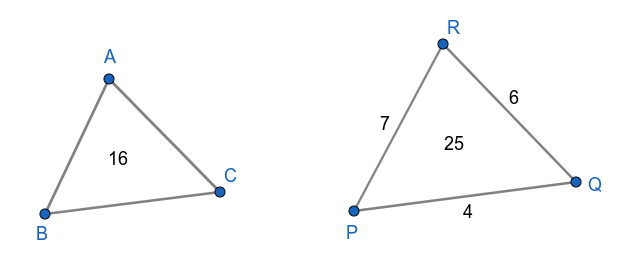$\triangle$ABC $\sim$ $\triangle$RPQ

$\Rightarrow$  $\left(\frac{AC}{QR}\right)^2=\frac{ar(\triangle\text{ABC})}{ar(\triangle\text{PQR})}$

$\Rightarrow$  $\left(\frac{AC}{6}\right)^2=\frac{16}{25}$

$\Rightarrow$  $\frac{AC}{6}=\frac{4}{5}$

$\Rightarrow$  AC = 4.8 cm

Hence, the correct answer is Option B

Question 7: In $\triangle$ABC, D is a point on BC such that $\angle$BAD = $\frac{1}{2} \angle$ADC and $\angle$BAC = 77$^\circ$ and $\angle$C = 45$^\circ$. What is the measure of $\angle$ADB?

a) $77^\circ$

b) $64^\circ$

c) $58^\circ$

d) $45^\circ$

Solution: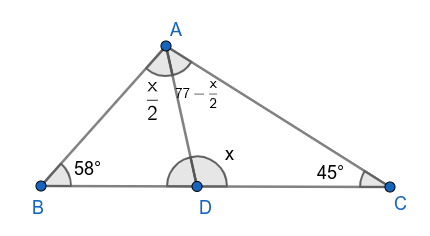$\angle$ADC = x

Given, $\angle$BAD = $\frac{1}{2} \angle$ADC

$\angle$BAD = $\frac{\text{x}}{2}$

$\angle$DAC = 77 – $\frac{\text{x}}{2}$

$\angle$ADC + $\angle$ACD + $\angle$DAC = 180$^\circ$

x + 45$^\circ$ + 77 – $\frac{\text{x}}{2}$ = 180$^\circ$

$\frac{\text{x}}{2}$ = 58$^\circ$

x = 116$^\circ$

$\angle$ADB = 180$^\circ$ – x

= 180$^\circ$ – 116$^\circ$

= 64$^\circ$

Hence, the correct answer is Option B

Question 8: The area of a triangular plot having sides 12 m, 35 m and 37 m is equal to the area of a rectangular field whose sides are in the ratio 7 : 3. The perimeter (in m) of the field is:

a) $20\sqrt{10}$

b) $20\sqrt{5}$

c) $24\sqrt{10}$

d) $24\sqrt{5}$

Solution:

Perimeter of triangular plot = 12 + 35 + 37 = 84 m

Half of the perimeter = s = 42 m

Area of the triangular plot = $\sqrt{42\left(42-12\right)\left(42-35\right)\left(42-37\right)}$

= $\sqrt{42\left(30\right)\left(7\right)\left(5\right)}$

= 210 cm$^2$

The area of a triangular plot is equal to the area of a rectangular field.

Area of a rectangular field = 210 cm$^2$

Sides of rectangular filed are in the ratio 7:3.

Let the sides of the rectangular field are 7p and 3p respectively.

7p x 3p = 210

p$^2$ = 10

p = $\sqrt{10}$

Perimeter of the rectangular field = 2(7p + 3p)

= 20p

= 20$\sqrt{10}$ cm

Hence, the correct answer is Option A

Question 9: In triangle PQR, points E and F are on sides PQ and PR respectively such that EF is parallel to QR. If PE = 2 cm and EQ = 3 cm, then area($\triangle$ PQR) : area($\triangle$ PEF) is equal to:

a) 3 : 2

b) 25 : 4

c) 5 : 2

d) 9 : 4

Solution: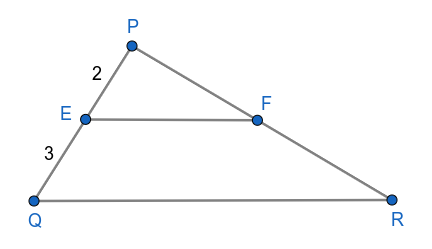$\triangle$PQR and $\triangle$PEF are similar triangles.

$\Rightarrow$  $\frac{\text{Area of triangle PQR}}{\text{Area of triangle PEF}}$ = $\left(\frac{PQ}{PE}\right)^2$

$\Rightarrow$  $\frac{\text{Area of triangle PQR}}{\text{Area of triangle PEF}}$ = $\left(\frac{PE+EQ}{PE}\right)^2$

$\Rightarrow$ $\frac{\text{Area of triangle PQR}}{\text{Area of triangle PEF}}$ = $\left(\frac{2+3}{2}\right)^2$

$\Rightarrow$ $\frac{\text{Area of triangle PQR}}{\text{Area of triangle PEF}}$ = $\frac{25}{4}$

$\Rightarrow$  Area of triangle PQR : Area of triangle PEF = 25:4

Hence, the correct answer is Option B

Question 10: The angles of a triangle are in AP (arithmetic progression). If measure of the smallest angle is $50^\circ$ less than that of the largest angle, then find the largest angle (in degrees).

a) 90

b) 85

c) 80

d) 75

Solution:

The angles of triangle are in AP (arithmetic progression).

Let the angles are a, a+r, a+2r.

Measure of the smallest angle is $50^\circ$ less than that of the largest angle.

a = a + 2r – $50^\circ$

2r = $50^\circ$

r = $25^\circ$

Sum of the angles of triangle = $180^\circ$

a + a + r + a + 2r = $180^\circ$

3a + 3r = $180^\circ$

3a + $75^\circ$ = $180^\circ$

3a = $105^\circ$

a = $35^\circ$

Largest angle of triangle = a + 2r = $35^\circ$ + $50^\circ$ = $85^\circ$

Hence, the correct answer is Option B

Question 11: Triangle ABC is an equilateral triangle. D and E are points on AB and AC respectively such that DE is parallel to BC and is equal to half the length of BC. If AD + CE + BC = 30 cm, then find the perimeter (in cm) of the quadrilateral BCED.

a) 37.5

b) 25

c) 45

d) 35

Solution: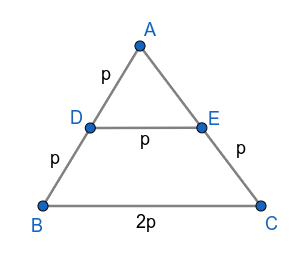Triangle ABC is an equilateral triangle.

Let the length of BC = 2p

BC = AB = AC = 2p

DE is equal to half the length of BC.

Triangle ABC and triangle ADE are similar triangles.

$\Rightarrow$  $\frac{AD}{AB}=\frac{DE}{BC}$

$\Rightarrow$  $\frac{AD}{AB}=\frac{p}{2p}$

$\Rightarrow$  $AD=\frac{1}{2}AB$

$\Rightarrow$  $AD=\frac{1}{2}\times2p$

$\Rightarrow$  AD = p

Similarly, AE = p

and EC = AC – AE = 2p – p = p

AD + CE + BC = 30 cm

p + p + 2p = 30

4p = 30

p = $\frac{15}{2}$ cm

Perimeter of the quadrilateral BCED = BD + DE + CE + BC

= p + p + p + 2p

= 5p

= $5\times\frac{15}{2}$

= 37.5 cm

Hence, the correct answer is Option A

Question 12: The side BC of a triangle ABC is extended to the point D. If $\angle$ACD = $132^\circ$ and $\angle$B = $\frac{4}{7} \angle$A, then the measure of $\angle$A is

a) $60^\circ$

b) $50^\circ$

c) $84^\circ$

d) $80^\circ$

Solution: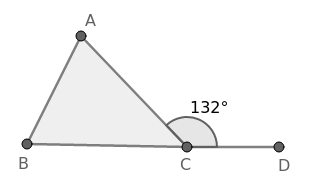Given, $\angle$ACD = $132^\circ$  and  $\angle$B = $\frac{4}{7} \angle$A

In $\triangle$ABC,

$\angle$ACD is the external angle at C which is equal to the sum of opposite angles A and B.

$\Rightarrow$  $\angle$ACD = $\angle$A + $\angle$B

$\Rightarrow$  $132^\circ$ = $\angle$A + $\frac{4}{7} \angle$A

$\Rightarrow$  $132^\circ$ = $\frac{11}{7} \angle$A

$\Rightarrow$  $\angle$A = $84^\circ$

Hence, the correct answer is Option C

Question 13: In a $\triangle$ABC, $\angle$BAC = $90^\circ$ and AD is perpendicular to BC where D is a point on BC. If BD = 4 cm and CD = 5 cm,then the length of AD is equal to:

a) $5 \sqrt 2$ cm

b) $2 \sqrt 5$ cm

c) 6 cm

d) 4.5 cm

Solution: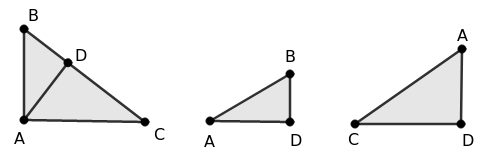In $\triangle$ABC,

$\angle$A = 90$^\circ$

$\Rightarrow$  $\angle$B = 90$^\circ$ – $\angle$C

$\Rightarrow$  $\angle$ADC = 90$^\circ$

In $\triangle$ADC,

$\angle$ADC = 90$^\circ$

$\Rightarrow$  $\angle$DAC = 90$^\circ$ – $\angle$C

In $\triangle$ADB and $\triangle$CDA,

$\angle$B = $\angle$DAC = 90$^\circ$ – $\angle$C

$\angle$BDA = $\angle$ADC = 90$^\circ$

Two angles are equal for both the triangles, $\triangle$ADB is similar to $\triangle$CDA

Ratio of respective sides are equal in both the triangles

$\Rightarrow$  $\frac{BD}{AD}=\frac{AD}{CD}$

$\Rightarrow$  AD$^2$ = BD.CD

$\Rightarrow$  AD$^2$ = 4 x 5

$\Rightarrow$  AD$^2$ =  20

$\Rightarrow$  AD = $2 \sqrt 5$ cm

Hence, the correct answer is Option B

Question 14: ABC is a right angled triangle, right angled at A. A circle is inscribed in it. The lengths of two sides containing the right angle are 48 cm and 14 cm. The radius of the inscribed circle is:

a) 4 cm

b) 8 cm

c) 6 cm

d) 5 cm

Solution: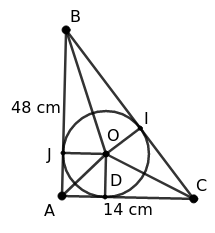Using the pythagoras theorem,

BC$^2$ = AB$^2$ + AC$^2$

$\Rightarrow$  BC$^2$ = 48$^2$ + 14$^2$

$\Rightarrow$  BC$^2$ = 2304 + 196

$\Rightarrow$  BC$^2$ = 2500

$\Rightarrow$  BC = 50 cm

Let the radius of the circle = r

$\Rightarrow$  OD = OI = OJ = r

AB, BC, AC are tangents to the circle

Area of $\triangle$ABC = Area of $\triangle$OAC + Area of $\triangle$OBC + Area of $\triangle$OAB

$\Rightarrow$  $\frac{1}{2}$ x 48 x 14 = $\frac{1}{2}$ x AC x OD + $\frac{1}{2}$ x BC x OI + $\frac{1}{2}$ x AB x OJ

$\Rightarrow$  336 = $\frac{1}{2}$ x 14 x r + $\frac{1}{2}$ x 50 x r + $\frac{1}{2}$ x 48 x r

$\Rightarrow$  336 = 7r + 25r + 24r

$\Rightarrow$  56r = 336

$\Rightarrow$  r = 6 cm

$\therefore\$Radius of the inscribed circle = 6 cm

Hence, the correct answer is Option C

Question 15: In an isosceles triangle ABC with AB = AC and AD is perpendicular to BC, if AD = 6 cm and the perimeter of $\triangle$ABC is 36 cm, then the area of $\triangle$ABC is:

a) 54 $cm^2$

b) 64 $cm^2$

c) 45 $cm^2$

d) 48 $cm^2$

Solution: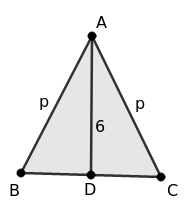Given, AB = AC

Let AB = AC = p

Perimeter of $\triangle$ABC = 36 cm

$\Rightarrow$  AB + AC + BC = 36

$\Rightarrow$  p + p + BC = 36

$\Rightarrow$  BC = 36 – 2p

Since AB = AC and AD is perpendicular to BC

AD will be the perpendicular bisector which bisects BC

$\Rightarrow$  BD = CD = $\frac{36-2p}{2}$ = 18 – p

In $\triangle$ADB,

AB$^2$ = BD$^2$ + AD$^2$

$\Rightarrow$  p$^2$ = (18 – p)$^2$ + 6$^2$

$\Rightarrow$  p$^2$ = 324 + p$^2$ – 36p + 36

$\Rightarrow$  36p = 360

$\Rightarrow$  p = 10

BC = 36 – 2p = 36 – 20 = 16

$\therefore\$Area of the triangle = $\frac{1}{2}$ x AD x BC = $\frac{1}{2}$ x 6 x 16 = 48 $cm^2$

Hence, the correct answer is Option D

Question 16: ABC and BDE are two equilateral triangles such that D is the mid-point of BC. If the area of triangle ABC is 136 cm$^2$,
then the area of triangle BDE is equal to:

a) $36 cm^2$

b) $38 cm^2$

c) $24 cm^2$

d) $34 cm^2$

Solution: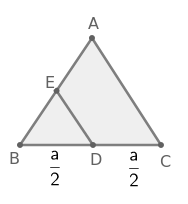Let the side of the equilateral triangle ABC = a

$\Rightarrow$  BC = a

D is the mid-point of BC

$\Rightarrow$  BD = $\frac{a}{2}$

Side of the equilateral triangle BDE = $\frac{a}{2}$

Given, Area of the equilateral triangle ABC = 136 cm$^2$

$\Rightarrow$  $\frac{\sqrt{3}}{4}a^2=136$

$\therefore\$Area of the equilateral triangle BDE = $\frac{\sqrt{3}}{4}\left(\frac{a}{2}\right)^2$

$=\frac{\sqrt{3}}{4}\times\frac{a^2}{4}$

$=\frac{1}{4}\times\frac{\sqrt{3}a^2}{4}$

$=\frac{1}{4}\times136$

$=$ 34 cm$^2$

Hence, the correct answer is Option D

Question 17: The side MN of $\triangle$LMN is produced to X. If $\angle$LNX = $117^\circ$ and $\angle$M = $\frac{1}{2} \angle$L, then $\angle$L is:

a) $78^\circ$

b) $76^\circ$

c) $77^\circ$

d) $75^\circ$

Solution: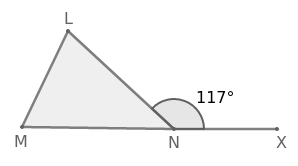Given, $\angle$LNX = $117^\circ$ and $\angle$M = $\frac{1}{2} \angle$L

In $\triangle$LMN,

The exterior angle LNX is equal to the sum of the opposite interior angles L and M.

$\Rightarrow$  $\angle$LNX = $\angle$L + $\angle$M

$\Rightarrow$  $117^\circ$ = $\angle$L + $\frac{1}{2} \angle$L

$\Rightarrow$  $117^\circ$ = $\frac{3}{2} \angle$L

$\Rightarrow$  $\angle$L =  $78^\circ$

Hence, the correct answer is Option A

Question 18: The centroid of an equilateral $\triangle$XYZ is L. If XY = 12 cm, then the length of XL (in cm), is:

a) $5 \sqrt 3$

b) $2 \sqrt 3$

c) $4 \sqrt 3$

d) $3 \sqrt 3$

Solution: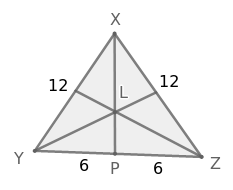In the equilateral traingle XYZ,  XY = 12 cm

$\Rightarrow$  YZ = XZ = 12 cm

The median XP bisects YZ at P and also perpendicular to YZ in the equilateral triangle.

$\Rightarrow$  YP = PZ = 6 cm   and  XP$\bot\$YZ

The centroid L divides the median XP in the ratio of 2 : 1

$\Rightarrow$ XL : PL = 2 : 1

$\Rightarrow$ XL = 2PL ………….(1)

In $\triangle$XPZ,

XP$^2$ + PZ$^2$ = XZ$^2$

$\Rightarrow$ XP$^2$ + 6$^2$ = 12$^2$

$\Rightarrow$ XP$^2$ + 36 = 144

$\Rightarrow$  XP$^2$ = 108

$\Rightarrow$  XP = $6\sqrt{3}$

$\Rightarrow$  XL + PL = $6\sqrt{3}$

$\Rightarrow$  2PL + PL = $6\sqrt{3}$

$\Rightarrow$  3PL = $6\sqrt{3}$

$\Rightarrow$  PL = $2\sqrt{3}$

$\Rightarrow$  XL = 2PL = $4\sqrt{3}$

Hence, the correct answer is Option C

Question 19: The measure of one of the exterior angles of a triangle is twice one of the interior opposite angles and the measure of the other interior opposite angles is 60$^\circ$. The triangle is a/an:

a) equilateral triangle

b) right triangle

c) scalene triangle

d) isosceles triangle

Solution:

Given, two interior angles of the triangle is 60$^\circ$.

$\Rightarrow$ The third interior angle must be 60$^\circ$ as the sum of the interior angles is 180$^\circ$.

$\therefore$The triangle is an equilateral triangle.

Hence, the correct answer is Option A

Question 20: In $\triangle$ABC, AB = AC, and $\angle$BAC is 50$^\circ$. Then $\angle$ABC and $\angle$BCA are, respectively:

a) $70^\circ$ and $75^\circ$

b) $65^\circ$ and $65^\circ$

c) $50^\circ$ and $55^\circ$

d) $55^\circ$ and $55^\circ$

Solution:

In $\triangle$ABC,  AB = AC

Angles opposite to equal sides in triangle are equal.

$\Rightarrow$ $\angle$BCA = $\angle$ABC

Let $\angle$ABC = $\angle$BCA = x

In $\triangle$ABC,

$\angle$ABC + $\angle$BCA + $\angle$BAC = 180$^\circ$

$\Rightarrow$  x + x + 50$^\circ$ = 180$^\circ$

$\Rightarrow$  2x = 130$^\circ$

$\Rightarrow$  x = 65$^\circ$

$\therefore\$ $\angle$ABC = $\angle$BCA = 65$^\circ$

Hence, the correct answer is Option B

Question 21: In $\triangle$ABC, D is a point on BC. If $\frac{AB}{AC} = \frac{BD}{DC}$, $\angle$B = $75^\circ$ and $\angle$C = $45^\circ$ then $\angle$BAD is equal to:

a) $50^\circ$

b) $45^\circ$

c) $30^\circ$

d) $60^\circ$

Solution: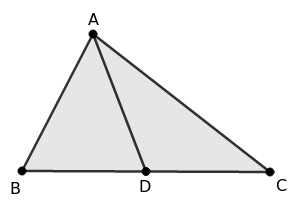Given, $\angle$B = $75^\circ$  and  $\angle$C = $45^\circ$

In $\triangle$ABC,

$\angle$A + $\angle$B + $\angle$C = $180^\circ$

$\Rightarrow$  $\angle$A + $75^\circ$ + $45^\circ$ = $180^\circ$

$\Rightarrow$  $\angle$A + $120^\circ$ = $180^\circ$

$\Rightarrow$  $\angle$A = $60^\circ$

Given, $\frac{AB}{AC} = \frac{BD}{DC}$

AD divides the side BC in the ratio of other two sides so AD is the angular bisector $\angle$A.

$\Rightarrow$  $\angle$BAD = $\frac{1}{2}\angle$A

$\Rightarrow$  $\angle$BAD = $\frac{60^{\circ}}{2}$

$\Rightarrow$  $\angle$BAD = $30^\circ$

Hence, the correct answer is Option C

Question 22: In $\triangle$ABC, E and D are points on sides AB and AC, respectively, such that $\angle$ABC = $\angle$ADE. If AE = 6 cm, AD = 4 cm
and EB = 4 cm, then the length of DC is:

a) 8 cm

b) 9.5 cm

c) 10 cm

d) 11 cm

Solution: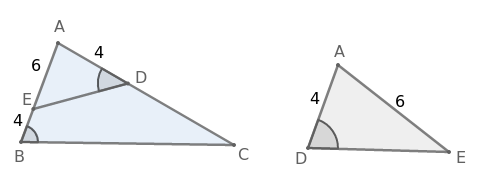In $\triangle$ABC and $\triangle$ADE,

$\angle$ABC = $\angle$ADE

$\angle$BAC = $\angle$DAE

So $\triangle$ABC is similar to $\triangle$ADE

$\Rightarrow$  $\frac{AB}{AC}=\frac{AD}{AE}$

$\Rightarrow$  $\frac{AE+EB}{AD+DC}=\frac{AD}{AE}$

$\Rightarrow$  $\frac{6+4}{4+DC}=\frac{4}{6}$

$\Rightarrow$  $\frac{10}{4+DC}=\frac{2}{3}$

$\Rightarrow$  4 + DC = 15

$\Rightarrow$  DC = 11 cm

Hence, the correct answer is Option D

Question 23: Triangle ABC is right angled at B and D is a point of BC such that BD = 5 cm, AD = 13 cm and AC = 37 cm. then find the length of DC in cm.

a) 25

b) 30

c) 5

d) 35

Solution: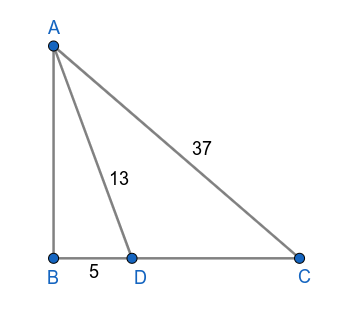From right angled triangle ABD,

AD$^2$ = AB$^2$ + BD$^2$

13$^2$ = AB$^2$ + 5$^2$

169 = AB$^2$ + 25

AB$^2$ = 144

AB = 12 cm

From right angled triangle ABC,

AC$^2$ = AB$^2$ + BC$^2$

37$^2$ = 12$^2$ + BC$^2$

1369 = 144 + BC$^2$

BC$^2$ = 1225

BC = 35

BD + DC = 35

5 + DC = 35

DC = 30 cm

Hence, the correct answer is Option B

Question 24: In $\triangle$ABC, $\angle$A = 50$^\circ$. If the bisectors of the angle B and angle C, meet at a point O, then $\angle$BOC is equal to:

a) $130^\circ$

b) $65^\circ$

c) $50^\circ$

d) $115^\circ$

Solution: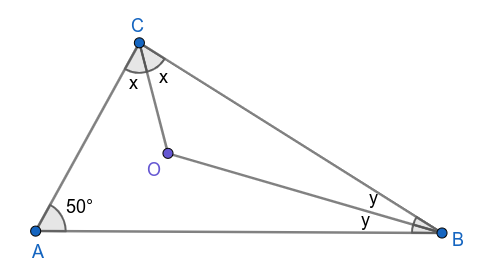BO is the bisector of angle B.

Let $\angle$OBC = $\angle$OBA = y

CO is the bisector of angle C.

Let $\angle$OCA = $\angle$OCB = x

From $\triangle$ABC,

$\angle$A + $\angle$B + $\angle$C = 180$^\circ$

50$^\circ$ + 2y + 2x = 180$^\circ$

2(x+y) = 130$^\circ$

x + y = 65$^\circ$……(1)

From $\triangle$OBC,

$\angle$OBC + $\angle$OCB + $\angle$BOC = 180$^\circ$

y + x + $\angle$BOC = 180$^\circ$

65$^\circ$ + $\angle$BOC = 180$^\circ$ [From (1)]

$\angle$BOC  = 115$^\circ$

Hence, the correct answer is Option D

Question 25: The perimeter of an isosceles triangle is 125 cm. If the base is 33 cm, find the length of the equal sides.

a) 46 cm

b) 34 cm

c) 32 cm

d) 42 cm

Solution: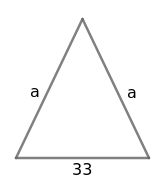Let length of the equal sides = a

Length of the base = 33 cm

Perimeter of isosceles triangle = 125 cm

$\Rightarrow$  a + a + 33 = 125

$\Rightarrow$  2a = 92

$\Rightarrow$  a = 46 cm

$\therefore\$Length of equal sides = 46 cm

Hence, the correct answer is Option A